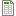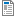# F7AMSBST - Biostatistics

Code Completion Credits Range Language
F7AMSBSCD Z,ZK 4 2P+2S English
Lecturer:
Vojtěch Kamenský, Vladimír Rogalewicz (guarantor), Jakub Ráfl
Tutor:
Vojtěch Kamenský
Supervisor:
Department of Biomedical Technology
Synopsis:

The course focuses on methods of statistical analysis designed primarily for medical research and clinical evaluation of medical devices. Students will be introduced to clinical research methodology, clinical study design and then to commonly used methods of processing and testing clinical data.

Requirements:

Requirements for the assessment:

1. Required attendance in seminars (max. 2 absences).

2. The student is required to be prepared for seminars in advance, deliver obligatory homework, and successfully pass the entrance test in the beginning of each seminar, student can be excluded from the particular seminar and considered absent, if he fails in these duties.

3. Student is required to pass the seminar test at the level of at least 50%

Requirements for the exam:

1. Passing the assessment.

The exam is oral, where the student draws on questions covering the topics of lectures. In order for a student to be admitted to the oral exam, they must have the requirements of assessment and write the exam test consisting of numerous examples for min. 50%

Syllabus of lectures:

Statistics, biostatistics, statistical calculations in the R programming language. Data processing. Graphical analysis. Introduction to probability. Random variable distribution. Random samples. Principles of statistic thinking. Estimation and hypothesis testing. Parametrical and non-parametrical test in normal distribution. T-tests. Binomial distribution and tests. Analysis of variance. Contingency tables. Correlation and regression.

Syllabus of tutorials:

Statistics, biostatistics, statistical calculations in the R programming language. Data processing. Graphical analysis. Introduction to probability. Random variable distribution. Random samples. Principles of statistic thinking. Estimation and hypothesis testing. Parametrical and non-parametrical test in normal distribution. T-tests. Binomial distribution and tests. Analysis of variance. Contingency tables. Correlation and regression.

Study Objective:

The aim of the study is to familiarize the student with the basic methods used in research design, its implementation, data processing, evaluation and interpretation.

Study materials:

Required:

CHATFIELD, Christopher. Statistics for technology: a course in applied statistics. 3rd ed. (rev.). Boca Raton, Fla.: Chapman &amp; Hall/CRC, 1998. ISBN 978-0-412-25340-9

Recommended:

SOCR. The Probability and Statistics EBook. Los Angeles: Statistics Online Computational Resource. 2014. (Volně dostupné na: http://wiki.stat.ucla.edu/socr/index.php/EBook)

Note:
The course is a part of the following study plans:

Lectures:
AttachmentSize1. Block of lectures: Introduction to biostatistics839.13 KB2. Block of lectures: Descriptive statistics509.85 KB3. Block of lectures: Tes of hypothesis 1406.81 KB4. Block of lectures: Tes of hypothesis 2398.97 KB5. Block of lectures: Tes of hypothesis 3361.92 KB

Exercises:
AttachmentSize1. Block of exercise: Introduction to R154.7 KB1. Block of exercise: Dataset33 KB2. Block of exercise: Confidence intervals88.29 KB5. Block of exercise: Tes of hypothesis in R14.09 KB

Others:
AttachmentSizeStatistical tables416.71 KB